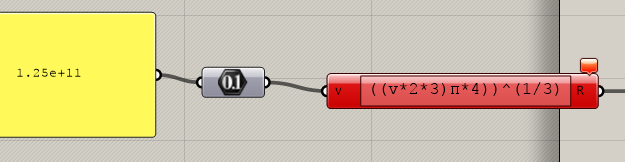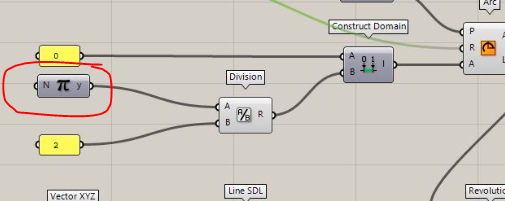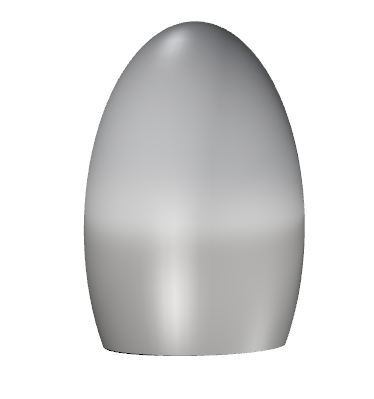# Half hemisphere - same volume as box

Hello,
I am trying to make an enclosed hemisphere
How do I make the base plane.
Also, how to calculate the volume of the same.

Arya

AryaSphere.gh (9.1 KB)

In regular Rhino, not Grasshoper, I would use Cap to cap the bottom and create a solid, and then Volume to calculate the volume.

Added: An alternative to Cap to create a solid is PlanarSrf followed by Join.

Hello David,

I tried using cap and volume for sphere.I need to make the two solids, a cube and a hemisphere with equal volume.Volume of the cube is 1.25E+11, I can’t achieve the same for the sphere.Have to bake it every time to check the volume.Therefore was asking if it could be done in gh.
Also even if the sphere is larger than the cube the volume is coming out to be lesser everytime.

For cube

For sphere

Arya

You can just do it mathematically:

V = 4/3 * Pi * r^3
Where V = volume and r = radius

So solving for r:
r = ((V * 3) / (4 * Pi))^(1/3)

In your case you want half a sphere so you need to multiply the volume by 2

r = ((2 * V * 3) / (4 * Pi))^(1/3)

You dont need GH, but if you do want to use it, it would look like this:

1 Like

Pufferfish has a handy scale to volume component which will scale any closed geometry to a desired volume, either proportionally or non proportionally. Has one for scaling to area too.

4 Likes

Hi
Why am I getting an error hereSphere.gh (8.5 KB)

Hi seghierkhaled,Which component is this

it’s Pi: Math >> Util

Done!

Thanks so much.
Arya

Thank you so muchArya

Can the same process be used to make an ellipse.
A half ellipse.
Which component to use in case of an ellipse.

calculate the volume of any shape by volume component and than cube root to create the box

or you mean create ellipse instead of cube ?

Yes an ellipse of the same volume as of the cube.

to create elipsoid i use sphere and scale ; but how to create elipsoid have the same volume of the cube
i don’t know because the ellipsoid have 3 different rayons

use the component mentionned by Michael Pryor
pufferfish scale to volume
i don’t know if there a component to create an ellipsoid but i created it based on sphere and scale
than scale to volume to get ellipsoid have the same volume of the cube

vol.gh (13.8 KB)

1 Like

Why am I getting an error here

The expression is not written the same as in my previous post. You are missing a division and brackets.

Yes I tried it too.
The component scales a sphere non uniformly.
Sorry to bother you I have one more question.
Can this kind of a closed sphere be made in grasshopper with the ellipse output, where I specify that I slice my ellipse upto certain height.
The output must be a closed geometry.Yes its working.Thanks a lot Adam.